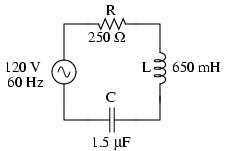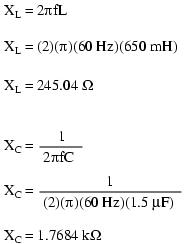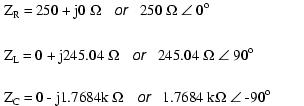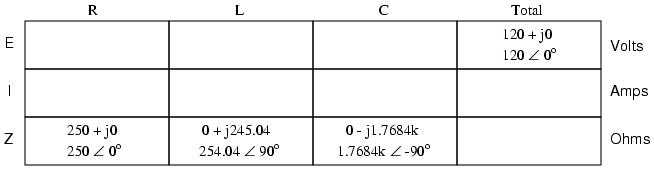Home   |  Schematics |  Products |  Tutorials  |  Datasheets  |  Robotics   |   Download    |   Link Exchange

 » Experiment » Calculator/Converters » Radio » Newsletter » Associations and Societies » Component Manufacturers

 Electronics Symentics A B C D E F G H I J K L M N O P Q R S T U V W X Y Z

 Reactance and Impedance RLC

 Let's take the following example circuit and analyze it:The first step is to determine the reactances (in ohms) for the inductor and the capacitor.The next step is to express all resistances and reactances in a mathematically common form: impedance. Remember that an inductive reactance translates into a positive imaginary impedance (or an impedance at +90 degrees), while a capacitive reactance translates into a negative imaginary impedance (impedance at -90 degrees). Resistance, of course, is still regarded as a purely "real" impedance (polar angle of 0 degrees):Now, with all quantities of opposition to electric current expressed in a common, complex number format (as impedances, and not as resistances or reactances), they can be handled in the same way as plain resistances in a DC circuit. This is an ideal time to draw up an analysis table for this circuit and insert all the "given" figures (total voltage, and the impedances of the resistor, inductor, and capacitor).Unless otherwise specified, the source voltage will be our reference for phase shift, and so will be written at an angle of 0 degrees. Remember that there is no such thing as an "absolute" angle of phase shift for a voltage or current, since it's always a quantity relative to another waveform. Phase angles for impedance, however (like those of the resistor, inductor, and capacitor), are known absolutely, because the phase relationships between voltage and current at each component are absolutely defined. Notice that I'm assuming a perfectly reactive inductor and capacitor, with impedance phase angles of exactly +90 and -90 degrees, respectively. Although real components won't be perfect in this regard, they should be fairly close. For simplicity, I'll assume perfectly reactive inductors and capacitors from now on in my example calculations except where noted otherwise.